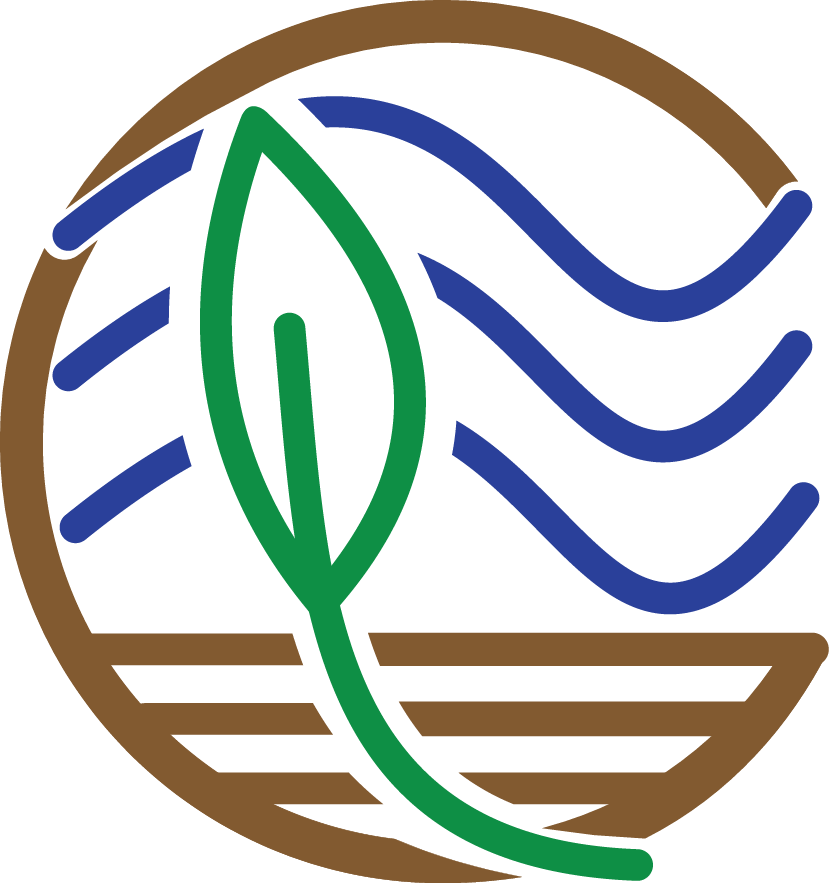Coastal CarbonChallenge Exercises

# Challenge ExercisesNow is time to put what you learned to the test with some challenges. Below are a few tasks for you to conduct in order to practice the commands you just learned. Your challenge is to use what you know about mutate, group_by and summarize, filter, and plotting in ggplot to complete the following tasks. Struggle builds character, but if you need a hint or want to check your answer, click the corresponding Hint and Solution buttons below.

1. Calculate organic matter density from bulk density and fraction organic matter. What is the average organic matter density in natural (impact_code = Nat) versus restored (impact_code = Res) sites?

First, We'll need to create a new column (organic matter density) that uses data from existing columns...sound familiar? Then, we'll need to combine data from two different data sets: depthseries and impact. Finally, we'll need to filter data to just the cores obtained from sites with the specified impact codes, and for each of these impact codes find the summary stat (mean) of our calculated column, organic matter density. We won't be able to correctly calculate our averages if there are any NA values for organic matter density, however. Give it a try!

# First, we'll need to calculate organic matter density. We did this earlier in the tutorial as well
depthseries_data_with_organic_matter_density <- depthseries_data %>%
mutate(organic_matter_density = dry_bulk_density * fraction_organic_matter)
# Now, we'll join together the depthseries and impact data
depthseries_with_omd_and_impact <- depthseries_data_with_organic_matter_density %>%
left_join(impact_data)
# We won't be able to summarize data if there are any NA values
depthseries_with_omd_and_impact <- depthseries_with_omd_and_impact %>%
filter(!is.na(organic_matter_density))

# Summarize for natural sites
depthseries_with_omd_and_impact %>%
filter(impact_code == "Nat") %>%
summarize(mean(organic_matter_density))

# Summarize for restored sites
depthseries_with_omd_and_impact %>%
filter(impact_code == "Res") %>%
summarize(mean(organic_matter_density))
# Organic matter density is slightly higher in natural sites than restored sites.


1. Plot a histogram of carbon density from 0 to 5 cm.

We will need to first subset to just the data we want: the depth series observations from a depth less than or equal to 5 cm. To do so, we'll need to group depth series observations by what core they are from, and then filter to just those from our desired depths. You will probably want to create a new object from our selected data. Then it's just as simple as constructing a histogram plot of our selected data. Check out the ggplot2 cheat sheet to help with this: https://www.rstudio.com/wp-content/uploads/2015/03/ggplot2-cheatsheet.pdf

# Filter for just data collected at depths of 5 cm or less
depthseries_data_shallow <- depthseries_data_with_carbon_density %>%
filter(depth_max <= 5)
# Plot the data as a histogram
depth_weighted_average_plot <- ggplot(depthseries_data_shallow, aes(carbon_density)) +
geom_histogram(bins = 100)
depth_weighted_average_plot


1. For each core with a maximum depth greater than or equal to 50 cm, calculate the average carbon density.

This is a tough one. Like the last challenge, we'll need to subset to just the cores we want. This time, we'll then need to acquire a summary stat for each core: the maximum value of the depth_max column. We want to filter to just cores that have such a value greater than or equal to 50...but we also have to make sure we retain ALL of the depth series observations for these long cores, including the observations at depths less than 50 cm. Once we have successfully accomplished this, we know the drill: calculate the average carbon density of the data grouped by each core.

# There are several methods by which you can narrow our selection of cores to just those that we want:
# Solution 1
depthseries_max_depths <- depthseries_data_with_carbon_density %>%
group_by(core_id) %>%
summarize(max_depth = max(depth_max))
# Create a new column, which contains the maximum depth sampled in each core
depthseries_data_long_cores <- depthseries_data_with_carbon_density %>%
left_join(depthseries_max_depths) %>%
# Join our depthseries data to the dataset that contains maximum depths
filter(max_depth >= 50)  # Select for only cores longer than 50 cm

# Another method is even more simple:
# Solution 2
depthseries_data_long_cores <- depthseries_data_with_carbon_density %>%
group_by(core_id) %>%
filter(max(depth_max) >= 50)

# Now calculate average carbon density
long_cores_average_carbon_density <- depthseries_data_long_cores %>%
group_by(core_id) %>%
summarize(mean(carbon_density))

long_cores_average_carbon_density


There is loads more that can be accomplished with our data using the tidyverse. But it’s easy to get overwhelmed by the many operations available, so the RStudio cheat sheets are immensely helpful:

We realize that learning a new coding language is a lot to take on: it’s a marathon, not a sprint. To help with that process, here are some additional resources that offer an introduction to R and RStudio: